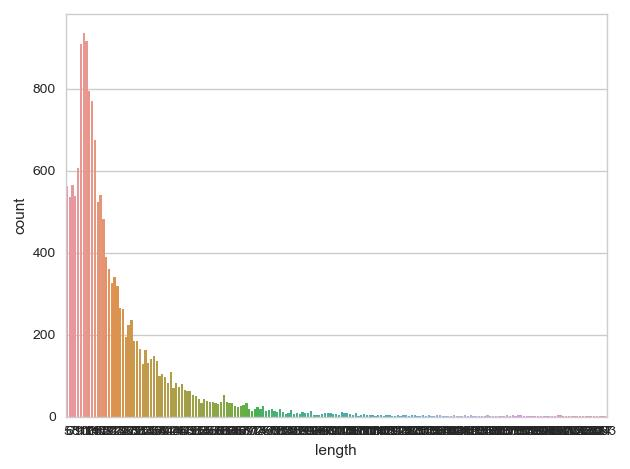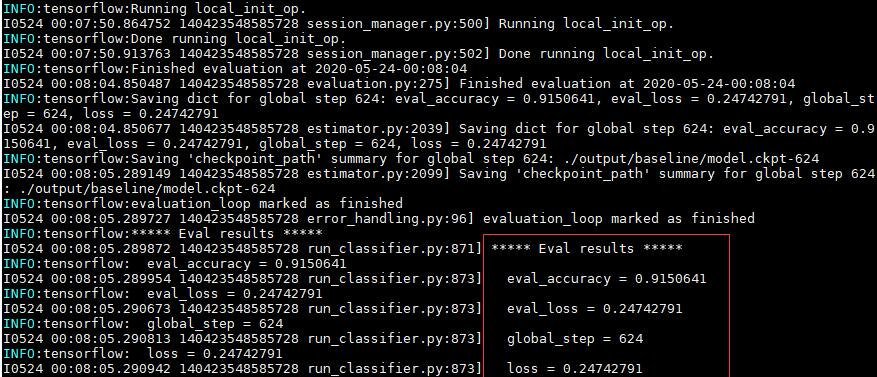【项目地址】：https://github.com/clvsit/bert-simple-use

## 准备工作

【数据】：案例使用的数据选择外卖情感极性评价数据集，这个数据集是我从 CSDN 的下载中找到的。

• 积极：
很快，好吃，味道足，量大


• 消极：
菜品质量好，味道好，就是百度的问题，总是用运力原因来解释，我也不懂这是什么原因，晚了三个小时呵呵厉害吧！反正订了就退不了，只能干等……• 将英文逗号替换为中文逗号，然后用英文逗号作为输入文本和标签的分隔符。
• 使用文章中没有出现的符号作为分隔符。

吃的挺好的,以后还会点别的&1

2:30送到,小伙伴们,看着办吧&0


【项目结构】：

model/
vocab.txt
bert_model.ckpt.meta
bert_model.ckpt.index
bert_model.ckpt.data-00000-of-00001
bert_config.json
data/
train.csv
dev.csv
test.csv
bert/
...
output/
export/
train.sh
predict.sh
predict.py
export.sh


## 训练和验证

（1）数据读取部分：找到 run_classifier.py 文件中的 DataProcessor(object) 类，我们要做的就是继承这个类，用来处理我们自己的数据。

• 修改读取文件的路径和名称。
def get_train_examples(self, data_dir):
return self._create_examples(

def get_dev_examples(self, data_dir):
return self._create_examples(

def get_test_examples(self, data_dir):
return self._create_examples(


• 修改标签函数。
def get_labels(self):
return ["0", "1"]


• 修改文件读取函数。
def _read_tsv(cls, input_file, quotechar=None):
with tf.gfile.Open(input_file, "r") as f:
lines = []
lines.append(line)
return lines


• 修改 _create_examples() 函数。
def _create_examples(self, lines, set_type):
examples = []
for (i, line) in enumerate(lines):
guid = "%s-%s" % (set_type, i)
if set_type == "test":
text_a = tokenization.convert_to_unicode(line)
label = "0"
else:
text_a = tokenization.convert_to_unicode(line)
label = tokenization.convert_to_unicode(line)

if label not in ["0", "1"]:
continue

examples.append(
InputExample(guid=guid, text_a=text_a, text_b=None, label=label))
return examples


_create_examples() 函数是修改的重点，在这里处理数据中各字段的读取，因为这是一个单句子任务，因此我们只需要 text_a 即可。

【完整代码】：

class EmotionProcessor(DataProcessor):

def get_train_examples(self, data_dir):
return self._create_examples(

def get_dev_examples(self, data_dir):
return self._create_examples(

def get_test_examples(self, data_dir):
return self._create_examples(

def get_labels(self):
return ["0", "1"]

with tf.gfile.Open(input_file, "r") as f:
lines = []
lines.append(line)
return lines

def _create_examples(self, lines, set_type):
examples = []
for (i, line) in enumerate(lines):
guid = "%s-%s" % (set_type, i)
if set_type == "test":
text_a = tokenization.convert_to_unicode(line)
label = "0"
else:
text_a = tokenization.convert_to_unicode(line)
label = tokenization.convert_to_unicode(line)

if label not in ["0", "1"]:
continue

examples.append(
InputExample(guid=guid, text_a=text_a, text_b=None, label=label))
return examples


processors = {
"emotion": EmotionProcessor,
}


【注意】：这里的 emotion 需要小写，因为 BERT 在读取 task_name 时进行了小写处理。

task_name = FLAGS.task_name.lower()



（2）模型配置部分：run_classifier.py 文件是一个命令行调用脚本文件，如果是在 linux 系统上，我们可以编写 bash 脚本，在这将训练和验证一起完成。

#! /bin/bash
export MODEL_DIR=model
export DATA_DIR=data

CUDA_VISIBLE_DEVICES=1 python bert/run_classifier.py \
--do_train=true \
--do_eval=true \
--data_dir=$DATA_DIR \ --vocab_file=$MODEL_DIR/vocab.txt \
--bert_config_file=$MODEL_DIR/bert_config.json \ --init_checkpoint=$MODEL_DIR/bert_model.ckpt \
--max_seq_length=128 \
--train_batch_size=32 \
--learning_rate=2e-5 \
--num_train_epochs=2.0 \
--output_dir=output


【注意】：

• 请根据自己机子的配置设置 train_batch_size 大小，以及 bert_config.json 中的配置内容。
• CUDA_VISIBVLE_DEVICES 指定要使用的显卡，如果只有一张显卡，设置为 0，即 CUDA_VISIBLE_DIVICES=0

sh train.sh## 模型推断

eval/
train.tf_record
model.ckpt-624.meta
model.ckpt-624.index
model.ckpt-624.data-00000-of-00001
model.ckpt-0.meta
model.ckpt-0.index
model.ckpt-0.data-00000-of-00001
graph.pbtxt
events.out.tfevents.xxx
eval_results.txt
eval.tf_record
checkpoint


#! /bin/bash
export MODEL_DIR=model
export DATA_DIR=data

CUDA_VISIBLE_DEVICES=1 python bert/run_classifier.py \
--do_predict=true \
--data_dir=$DATA_DIR \ --vocab_file=$MODEL_DIR/vocab.txt \
--bert_config_file=$MODEL_DIR/bert_config.json \ --init_checkpoint=output/model.ckpt-624 \ --output_dir=output  相比 train.sh 文件，将 do_traindo_eval 修改为 do_predict，并指定 init_checkpoint 为我们训练完成的模型文件。如果你想将推断结果存放到其他目录下，可以修改 output_dir 参数值。在这，我仍然将推断的结果存储到 output 目录下，此时可以看到多了 test_results.tsv 文件。 0.011303517 0.98869646 0.9418804 0.058119625 0.04432816 0.9556718 0.011732221 0.98826784 0.029930793 0.9700693 0.012002373 0.98799765 0.09023312 0.90976685 0.017287388 0.9827126 0.03125599 0.968744 0.015658164 0.9843418  该文件记录模型对各标签的预测概率值，例如第一条消极的概率为 0.011303517，积极的概率为 0.98869646，模型认为第一条评论是积极的。查看 test.csv 文件的第一条评论“菜量很大，味道也不错，师傅速度很快，好评～”，模型的预测是正确的。 ### 模型导出和使用 虽然我们可以直接使用 predict.sh 去做预测，但问题是我们需要将预测的数据做成 csv 文件，然后启动 predict.sh，能不能做成函数的形式，把输入传给一个函数，然后得到相应的结果？当然可以！ 首先，我们需要在 run_classifier.py 文件中新增导出的代码。 • 在代码的 flags 区域加上 export_dirdo_export flags.DEFINE_string( "export_dir", None, "The dir where the exported model will be written.") flags.DEFINE_bool( "do_export", False, "Whether to export the model.")  • 然后创建 serving_input_fn() 函数。 def serving_input_fn(): input_ids = tf.placeholder(tf.int32, [None, FLAGS.max_seq_length], name='input_ids') input_mask = tf.placeholder(tf.int32, [None, FLAGS.max_seq_length], name='input_mask') segment_ids = tf.placeholder(tf.int32, [None, FLAGS.max_seq_length], name='segment_ids') input_fn = tf.estimator.export.build_raw_serving_input_receiver_fn({ 'label_ids': tf.constant(0, tf.int32), 'input_ids': input_ids, 'input_mask': input_mask, 'segment_ids': segment_ids })() return input_fn  • 接着在 main() 函数中修改如下几处代码。 if not FLAGS.do_train and not FLAGS.do_eval and not FLAGS.do_predict and not FLAGS.do_export: raise ValueError( "At least one of do_train, do_eval or do_predict' must be True.")  • 最后，在 main() 函数的尾部加上导出相关的代码。 if FLAGS.do_export: estimator._export_to_tpu = False estimator.export_savedmodel(FLAGS.export_dir, serving_input_fn)  完成代码的修改后，接着把训练好的模型转换成 savedModel 形式，开始编写 export.sh 文件。 #! /bin/bash export MODEL_DIR=model export DATA_DIR=data CUDA_VISIBLE_DEVICES=1 python bert/run_classifier.py \ --task_name=Emotion \ --do_export=true \ --data_dir=$DATA_DIR \
--vocab_file=$MODEL_DIR/vocab.txt \ --bert_config_file=$MODEL_DIR/bert_config.json \
--init_checkpoint=output/model.ckpt-624 \
--output_dir=output
--export_dir=export


SavedModel written to: exported/temp-b'1590300832'/saved_model.pb


export 目录下多了 temp-b’1590300779’ 和 1590300832 的两个目录，其中 1590300832 是导出的 saveModel 以时间戳命名。

import tensorflow as tf
from bert import tokenization

def convert_single_example(query, label_list, max_seq_length, tokenizer):
"""Converts a single InputExample into a single InputFeatures."""
label_map = {}
for (i, label) in enumerate(label_list):
label_map[label] = i

tokens_a = tokenizer.tokenize(query)

if len(tokens_a) > max_seq_length - 2:
tokens_a = tokens_a[0:(max_seq_length - 2)]

tokens = []
segment_ids = []
tokens.append("[CLS]")
segment_ids.append(0)
for token in tokens_a:
tokens.append(token)
segment_ids.append(0)
tokens.append("[SEP]")
segment_ids.append(0)

input_ids = tokenizer.convert_tokens_to_ids(tokens)

while len(input_ids) < max_seq_length:
input_ids.append(0)
segment_ids.append(0)

return {
"input_ids": input_ids,
"segment_ids": segment_ids,
}

if __name__ == '__main__':
label_list = [0, 1]
predict_fn = tf.contrib.predictor.from_saved_model("exported/1590300832/")
tokenizer = tokenization.FullTokenizer(vocab_file="model/vocab.txt", do_lower_case=True)
feature = convert_single_example("菜量很大，味道也不错，师傅速度很快，好评～", label_list, 128, tokenizer)
prediction = predict_fn({
"input_ids": [feature['input_ids']],
"segment_ids": [feature['segment_ids']],
})
probabilities = prediction["probabilities"]
label = label_list[probabilities.argmax()]
print(probabilities)
print(label)



• 读取 saveModel：注意替换成自己模型的名称哦。
predict_fn = tf.contrib.predictor.from_saved_model("exported/1590300832/")

• 整理输入数据格式。
tokenizer = tokenization.FullTokenizer(vocab_file="model/vocab.txt", do_lower_case=True)
feature = convert_single_example("菜量很大，味道也不错，师傅速度很快，好评～", label_list, 128, tokenizer)

• 将输入数据传给预测函数，得到预测结果。
prediction = predict_fn({
"input_ids": [feature['input_ids']],
"segment_ids": [feature['segment_ids']],
})
`08-17
06-013万+
02-027693
07-07220
12-06818
01-201万+
11-231万+
11-229023
08-1110万+
05-066131
11-215511
12-26259
10-17499